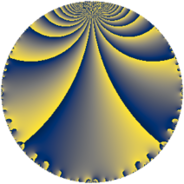Properties

 Label 24.3.bLevel $24$ Weight $3$ Character orbit 24.b Rep. character $\chi_{24}(19,\cdot)$ Character field $\Q$ Dimension $4$ Newform subspaces $1$ Sturm bound $12$ Trace bound $0$

Related objects

Defining parameters

 Level: $$N$$ $$=$$ $$24 = 2^{3} \cdot 3$$ Weight: $$k$$ $$=$$ $$3$$ Character orbit: $$[\chi]$$ $$=$$ 24.b (of order $$2$$ and degree $$1$$) Character conductor: $$\operatorname{cond}(\chi)$$ $$=$$ $$8$$ Character field: $$\Q$$ Newform subspaces: $$1$$ Sturm bound: $$12$$ Trace bound: $$0$$

Dimensions

The following table gives the dimensions of various subspaces of $$M_{3}(24, [\chi])$$.

Total New Old
Modular forms 10 4 6
Cusp forms 6 4 2
Eisenstein series 4 0 4

Trace form

 $$4 q + 2 q^{2} - 8 q^{4} - 6 q^{6} - 4 q^{8} + 12 q^{9} + O(q^{10})$$ $$4 q + 2 q^{2} - 8 q^{4} - 6 q^{6} - 4 q^{8} + 12 q^{9} + 12 q^{10} - 32 q^{11} - 12 q^{12} + 36 q^{14} - 8 q^{16} - 8 q^{17} + 6 q^{18} + 32 q^{19} + 72 q^{20} - 16 q^{22} + 36 q^{24} - 44 q^{25} - 96 q^{26} - 48 q^{28} - 60 q^{30} - 88 q^{32} + 44 q^{34} + 96 q^{35} - 24 q^{36} + 40 q^{38} + 120 q^{40} + 40 q^{41} + 84 q^{42} + 32 q^{43} + 64 q^{44} - 72 q^{46} + 96 q^{48} - 44 q^{49} - 118 q^{50} - 96 q^{51} - 48 q^{52} - 18 q^{54} - 168 q^{56} - 48 q^{57} + 156 q^{58} - 128 q^{59} - 96 q^{60} + 204 q^{62} - 32 q^{64} + 96 q^{65} + 48 q^{66} - 256 q^{67} + 112 q^{68} + 24 q^{70} - 12 q^{72} + 200 q^{73} - 120 q^{74} + 192 q^{75} - 16 q^{76} - 48 q^{78} - 96 q^{80} + 36 q^{81} - 124 q^{82} + 160 q^{83} - 24 q^{84} + 88 q^{86} + 32 q^{88} - 200 q^{89} + 36 q^{90} + 288 q^{91} + 96 q^{92} - 168 q^{94} - 24 q^{96} + 56 q^{97} + 170 q^{98} - 96 q^{99} + O(q^{100})$$

Decomposition of $$S_{3}^{\mathrm{new}}(24, [\chi])$$ into newform subspaces

Label Dim. $$A$$ Field CM Traces $q$-expansion
$a_{2}$ $a_{3}$ $a_{5}$ $a_{7}$
24.3.b.a $4$ $0.654$ 4.0.4752.1 None $$2$$ $$0$$ $$0$$ $$0$$ $$q+(\beta _{1}-\beta _{2})q^{2}+\beta _{2}q^{3}+(-2-\beta _{2}+\cdots)q^{4}+\cdots$$

Decomposition of $$S_{3}^{\mathrm{old}}(24, [\chi])$$ into lower level spaces

$$S_{3}^{\mathrm{old}}(24, [\chi]) \cong$$ $$S_{3}^{\mathrm{new}}(8, [\chi])$$$$^{\oplus 2}$$